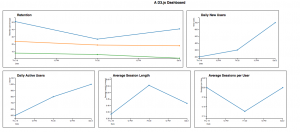# Building a lightweight, flexible D3.js dashboard (Part 2 of 3)(You can find the complete sourcecode for the below example here and an interactive example here. Click open in new window to see everything in its pre-determined size).

The first post in this series introduced the D3.js library and walked through the steps necessary to produce a very simple line graph. This post will build upon that tutorial to deliver a framework for programmatically creating charts for the different metrics present in a JSON dataset.

The dataset in the first post was extremely simple and not representative of what one would work with in a real dashboard. So we’ll add to it: we’ll create an actual JSON object containing multiple metrics over multiple days, aggregated over one dimension: country (and we’ll use just one country). The first thing we’ll do is create a helper function called getData to populate the dataset:

This function creates a JSON object called metric containing three days’ worth of metrics, aggregated under the dimension country. It then iterates through that object and populates the array data with elements: the first element is the country of the metrics, and the next elements are the days worth of metrics for that country. We only have one country in this example, but in the next post, we’ll explore a situation where multiple days worth of metrics are delivered for multiple countries. Data is an array containing one element, which contains an array of four elements: the country in question, and three days’ worth of metrics. (Note: the retention data is knowingly unrealistic. A normal retention graph should show Day 1 retention for today at 0, Day 7 retention for 4 days ago at 0, etc.)

The next step is creating a helper function which can create averages for a specific metric. You may notice that we include retention metrics and session metrics in the JSON object; these aren’t instructive as-is because they represents counts, so we should create averages based on new users (for retention) and total users and total sessions (for sessions). The helper function we’ll create is called createAverages:

createAverages takes one metric (one element of the data array) and returns it with averages creates for retention (where the retention metrics are recorded relative to the new users for that day) and sessions. You may notice that createAverages calls a function called safeDivide; this is because the SVG object will render all data points as NaN if it receives a single NaN value in graph data. To avoid that, the safeDivide returns 0 when any NaN values are input. The safeDivide function is:

Now we must modify two helper functions from the previous post: getMinObjectValue and getMaxObjectValue. Because we will want to plot more than one graph on each chart, we’ll have to adapt these helper functions to accept arrays: the metric object is now an array of data points, and we’ll also need to provide it with an array of object elements from which to take minimum and maximum values (to create the axes). We’ll modify them to look like this:

A few notes:

• When the value of the element is less than 1 (such as when we’re dealing with a percentage, like retention), we don’t want to return the floor or ceiling values of those, because they’ll default to the next higher or lower integers. So we test for values less than 1 and proceed accordingly.
• We can use bracket notation with an object element in javascript.

We’ll need to create a new helper function to access the data of an object when graphing our data points, since the JSON object doesn’t store dates as javascript Date objects (like in the previous post). This new helper function will be called getDate:

Now we’ll want to include all of our graphing code in an object so that we can create graphs easily. To do this, we’ll create a function called buildLineChart. This function takes a number of parameters: data (one array of data points), title (the title of the graph that we’ll append to the graph object), graph_metric (an array of metrics that we’ll plot on the graph), width (the width of the chart object), height (the height of the chart object), xaxislabel (the label we’ll append to the x axis of the chart), and yaxislabel (the label we’ll append to the y axis of the chart). This is the code for buildLineChart:

You’ll notice one fundamental difference between this function and the code from the first post: we loop through the graph_metric array and append data points for each set of metrics it contains. We also add something new from the last post: data labels (svg:title objects) for each data point that show natively upon mouseover. When our chart hosts multiple graphs (when graph_metric.length > 1), we include an additional line of text indicating which graph_metric that data point is a member of. We also create the colorscale array, which contains a number of color elements that we can apply to each graph in the chart (when we have more than one). This helps to differentiate between the graphs. The first two lines of code in the function clone metric so that it isn’t accessed by reference.

The last step in building our dashboard is creating the document.ready jQuery function to graph everything. We’ll create the data array, create the averaged elements, and then create five charts: Retention (containing graphs of day 1, day 7, and day 30 retention data points), Daily New Users, Daily Active Users, Average Session Length (averaged from the original data), and Average Sessions per User. The code for that is:

When these code fragments are combined and opened in a browser, they produce the following collection of charts (note that the Retention chart was provided with a larger width value than the rest of the charts):The next — and final — post in this series will explore a multi-dimensional dataset and provide a framework for dynamically browsing single and multi-dimension graphs.